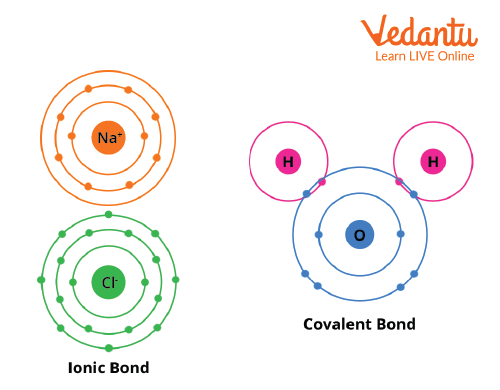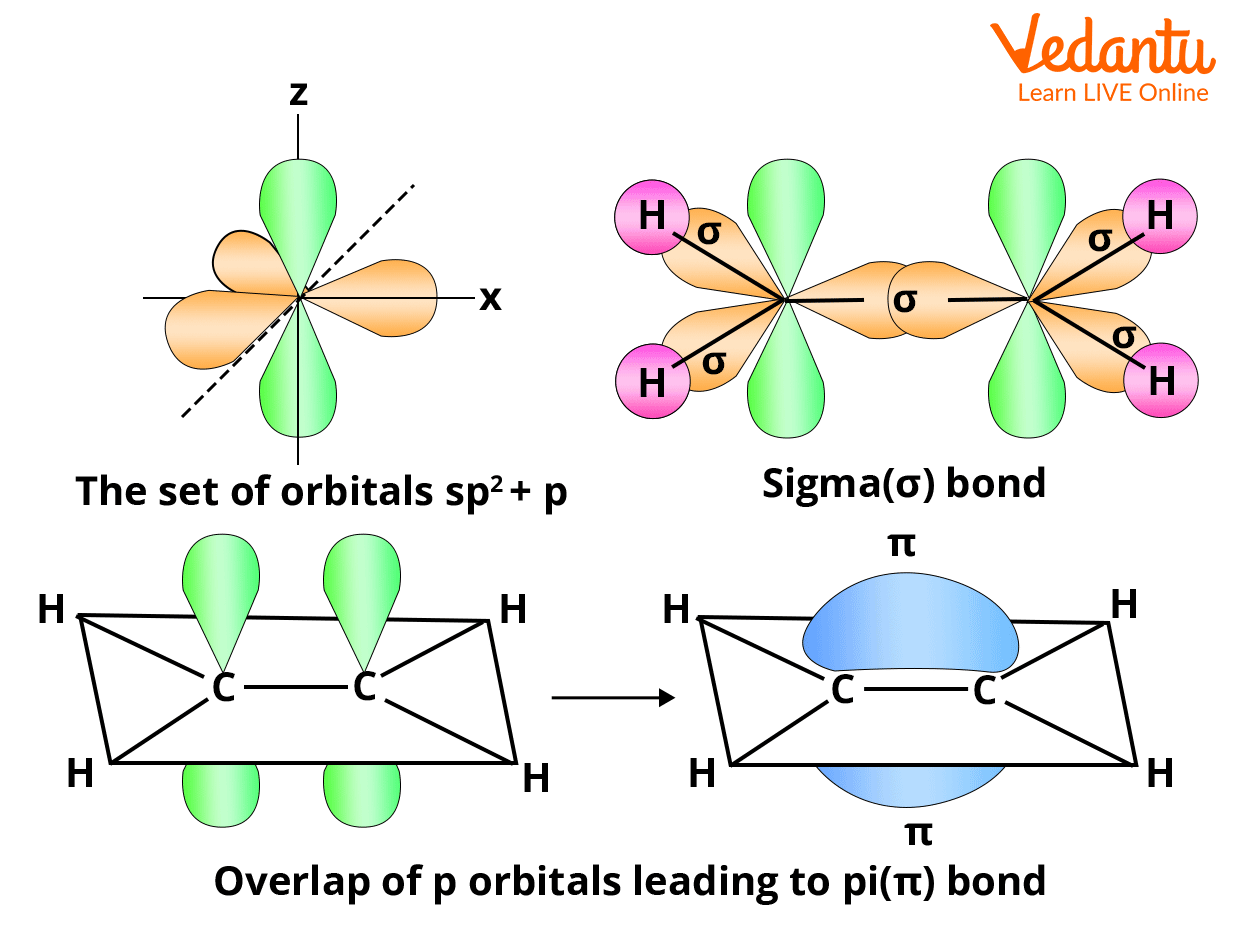Courses
Courses for Kids
Free study material
Free LIVE classes
More

# Calculation of Sigma and Pi Bonds for JEELIVE
Join Vedantu’s FREE Mastercalss

## Introduction to Bond Formation

To achieve a stable electronic arrangement, atoms either share, gain, or lose electrons. This results in a condition of minimal energy and the formation of a chemical bond. As a result, maximum stability is achieved. Covalent bonds are created when two atoms share electrons, and ionic bonds are generated when atoms lose or gain electrons. The complete transfer of valence electron(s) between atoms is referred to as ionic bonding. It is a form of chemical bond that produces two ions with opposite charges. The sharing of electrons between atoms is known as covalent bonding. This sort of bonding occurs between two atoms of the same element or elements in the periodic table that are close to each other. This bonding is most common between nonmetals.

Covalent bonds are most likely to form when atoms have similar electronegativities (affinities for electrons). Otherwise, if both atoms have the same affinity for electrons and neither has a great ability to donate them, they will share electrons to attain an octet configuration and become more stable. With a better understanding of bond formation and its types, we can find how to count sigma and pi bonds in any molecule.Covalent and Ionic bond

## Sigma Bonds

Head-on-head (same phase) overlap of atomic orbitals along the internuclear axis forms this sort of covalent bond. Due to the direct overlapping of the participating orbitals, sigma bonds are the strongest covalent bonds. The electrons that participate in a bond are referred to as sigma electrons.

A sigma bond (bond) is a bond produced by the end-to-end overlap of orbitals, with the electron density centered between the bonding atoms' nuclei.

The methane molecule, for example, has four C-H sigma bonds. The overlap of bonding orbitals along the internuclear axis forms this type of covalent bond.

## Pi bonds

Pi bonds are covalent chemical bonds that involve the lateral or sidewise overlapping of two lobes of one atomic orbital with two lobes of another atomic orbital from a different atom. The Greek letter π relates to the comparable symmetry of the pi bond and the p orbital, which is why pi bonds are sometimes written as π bonds'. Almost always, pi bonds are weaker than sigma bonds.

A single sigma bond and a single pi bond make up a double bond in most cases.Sigma and pi bonds

## How to Calculate Sigma and Pi Bonds?

In most organic compounds, all atom-to-atom bonds comprise one sigma bond each. If it is a single bond, only the sigma bond is present. Sigma and pi bonds, on the other hand, are found in multiple bonds (double and triple). Triple bonds have one sigma bond and two pi bonds, while double bonds have one sigma bond and one pi bond. The sigma and pi bonds in large organic molecules can be calculated out as follows:

## Sigma and Pi Bonds in Open Chain Alkenes

Pi and double bonds

In an unsaturated hydrocarbon having double bonds, we must count the number of carbon atoms (X) and hydrogen atoms (Y). For an aliphatic straight chain olefin, the formula for calculating the number of bonds or double bonds is:

$\mathrm{P}=\dfrac{2 \mathrm{X}-\mathrm{Y}}{2}+1$

Sigma bonds

The formula for calculating the number of sigma bonds for an aliphatic straight chain olefin is

S=X+Y−1

Single bonds

For an aliphatic straight chain olefin, the total number of single bonds is

$\mathrm{A}=\dfrac{3 \mathrm{Y}}{2}-2$

Example: Calculate the total number of sigma and pi bonds present in 2 butene.

C4H8

X=4 Y=8

by using formula

\begin{align} &P=\dfrac{2 \times 4-8}{2}+1=1 \\ &S=4+8-1=11 \\ &A=\dfrac{3 \times 8}{2}-2=10 \end{align}

The molecule contains one pi bond 11 sigma bond 10 single bonds.

## Calculation of Sigma and Pi Bonds in Cyclic Olefinic Hydrocarbon

Pi bonds

The formula for calculating the number of single bonds or double bonds is:

$P=\dfrac{2 X-Y}{2}$

Sigma bond

The formula for calculating  the number of sigma bonds for unsaturated cyclic  olefin is

S=X+Y

Single bond

For an unsaturated cyclic  olefin, the total number of single bonds is

$A=\dfrac{3Y}{2}$

Example: Calculate the ratio of sigma and pi bonds in benzene.

C6H6

X=6 Y=6

\begin{align} &P=\dfrac{12-6}{2}=3 \\ &S=6+6=12 \\ &A=\dfrac{18}{ 2}=9 \end{align}

Benzene has 3 pi,12 sigma and 9 single bonds.

## Conclusion

Covalent bonds are created when two atoms share electrons, and ionic bonds are generated when atoms lose or gain electrons. The complete transfer of valence electron(s) between atoms is referred to as ionic bonding. In most organic compounds, all atom-to-atom bonds comprise one sigma bond each. If it is a single bond, only then the sigma bond is present. Sigma and pi bonds, on the other hand, are found in multiple bonds. We further discussed how to find sigma and pi bonds in aliphatic and cyclic unsaturated olefinic compounds. Hence, with the help of these formulas, we can deduce the structure of organic compounds using their molecular formulas.

Last updated date: 21st Sep 2023
Total views: 132.3k
Views today: 4.32k

## FAQs on Calculation of Sigma and Pi Bonds for JEE

1. Define a coordinate bond.

A coordinate bond (also known as a dative covalent bond) is a type of connection (a shared pair of electrons) in which both electrons originate from the same atom. Two atoms sharing a pair of electrons make a covalent bond. As both the nuclei are attracted to the electron pair, the atoms are held together.

An electrophile forms coordinate connections with one or more nucleophiles arranged around it. To satisfy the octet rule, nucleophiles function as "ligands" by supplying two electrons per coordinate bond to the electrophile.

2. Define Hybridisation.

In chemistry, hybridisation is defined as the process of combining two atomic orbitals to create a new type of hybridised orbital. The intermixing of orbitals results in the formation of hybrid orbitals having different energies than their parent orbitals. Hybridisation is mostly carried out by atomic orbitals of the same energy level. Fully filled and half-filled orbitals, however, can both participate in this process if their energies are equivalent.

On the other hand, we can say that the concept of hybridisation is a development of the valence bond theory, and it aids in our understanding of bond formation, bond energies, and bond lengths.